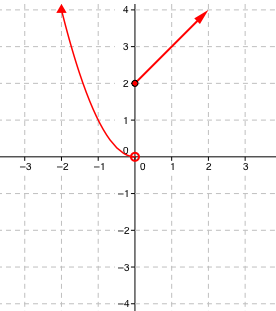### Home > CCAA8 > Chapter 10 Unit 11 > Lesson CCA: 10.2.5 > Problem10-87

10-87.

In Chapters 2 and 6 you saw some examples of piecewise graphs. At right is an equation for a piecewise graph.                          $f ( x ) = \left\{ \begin{array} { l l } { x + 2 } & { \text { if } x \geq 0 } \\ { x ^ { 2 } } & { \text { if } x < 0 } \end{array} \right.$

This equation states that for non-negative input values the top rule is used and for negative input values, the bottom rule is used.

For example, for an input of $x=3$, the output is $3+2=5$ and therefore the point $(3,5)$ is on the graph. However, for an input of $x=-2$, the output is $(-2)^2=4$ and therefore the point $(-2,4)$ is on the graph. Use various other input values and sketch a graph of this piecewise function.

Complete the table in the eTool below to create a graph of the piecewise function.
Click the link at right for the full version of the eTool: CCA 10-87 HW eTool